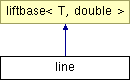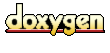Main Page   Class Hierarchy   Compound List   File List   Compound Members   File Members

# line Class Template Reference

Line (with slope) wavelet. More...

`#include <line.h>`

Inheritance diagram for line::List of all members.

## Protected Methods

void predict (T &vec, int N, transDirection direction)
Predict phase of line Lifting Scheme wavelet. More...

void update (T &vec, int N, transDirection direction)
Update step of the linear interpolation wavelet. More...

## Private Methods

double new_y (double y1, double y2)
Calculate an extra "even" value for the line wavelet algorithm at the end of the data series. More...

## Detailed Description

### template<class T> class line

Line (with slope) wavelet.

The wavelet Lifting Scheme "line" wavelet approximates the data set using a line with with slope (in contrast to the Haar wavelet where a line has zero slope is used to approximate the data).

The predict stage of the line wavelet "predicts" that an odd point will lie midway between its two neighboring even points. That is, that the odd point will lie on a line between the two adjacent even points. The difference between this "prediction" and the actual odd value replaces the odd element.

The update stage calculates the average of the odd and even element pairs, although the method is indirect, since the predict phase has over written the odd value.

You may use this source code without limitation and without fee as long as you include:

This software was written and is copyrighted by Ian Kaplan, Bear Products International, www.bearcave.com, 2001.

This software is provided "as is", without any warranty or claim as to its usefulness. Anyone who uses this source code uses it at their own risk. Nor is any support provided by Ian Kaplan and Bear Products International.

Please send any bug fixes or suggested source changes to:

```     iank@bearcave.com
```

Author:
Ian Kaplan

Definition at line 54 of file line.h.

## Member Function Documentation

 template double line::new_y ( double y1, double y2 )` [inline, private]`
 Calculate an extra "even" value for the line wavelet algorithm at the end of the data series. Here we pretend that the last two values in the data series are at the x-axis coordinates 0 and 1, respectively. We then need to calculate the y-axis value at the x-axis coordinate 2. This point lies on a line running through the points at 0 and 1. Given two points, x1, y1 and x2, y2, where ``` x1 = 0 x2 = 1 ``` calculate the point on the line at x3, y3, where ``` x3 = 2 ``` The "two-point equation" for a line given x1, y1 and x2, y2 is ``` . y2 - y1 (y - y1) = -------- (x - x1) . x2 - x1 ``` Solving for y ``` . y2 - y1 y = -------- (x - x1) + y1 . x2 - x1 ``` Since x1 = 0 and x2 = 1 ``` . y2 - y1 y = -------- (x - 0) + y1 . 1 - 0 ``` or ``` y = (y2 - y1)*x + y1 ``` We're calculating the value at x3 = 2, so ``` y = 2*y2 - 2*y1 + y1 ``` or ``` y = 2*y2 - y1 ``` Definition at line 125 of file line.h. Referenced by predict(). ```00126 { 00127 double y = 2 * y2 - y1; 00128 return y; 00129 } ```

 template void line::predict ( T & vec, int N, transDirection direction )` [inline, protected, virtual]`
 Predict phase of line Lifting Scheme wavelet. The predict step attempts to "predict" the value of an odd element from the even elements. The difference between the prediction and the actual element is stored as a wavelet coefficient. The "predict" step takes place after the split step. The split step will move the odd elements (bj) to the second half of the array, leaving the even elements (ai) in the first half ``` a0, a1, a1, a3, b0, b1, b2, b2, ``` The predict step of the line wavelet "predicts" that the odd element will be on a line between two even elements. ``` bj+1,i = bj,i - (aj,i + aj,i+1)/2 ``` Note that when we get to the end of the data series the odd element is the last element in the data series (remember, wavelet algorithms work on data series with 2n elements). Here we "predict" that the odd element will be on a line that runs through the last two even elements. This can be calculated by assuming that the last two even elements are located at x-axis coordinates 0 and 1, respectively. The odd element will be at 2. The new_y() function is called to do this simple calculation. Reimplemented from liftbase. Definition at line 169 of file line.h.```00170 { 00171 int half = N >> 1; 00172 double predictVal; 00173 00174 for (int i = 0; i < half; i++) { 00175 int j = i + half; 00176 if (i < half-1) { 00177 predictVal = (vec[i] + vec[i+1])/2; 00178 } 00179 else if (N == 2) { 00180 predictVal = vec; 00181 } 00182 else { 00183 // calculate the last "odd" prediction 00184 double n_plus1 = new_y( vec[i-1], vec[i] ); 00185 predictVal = (vec[i] + n_plus1)/2; 00186 } 00187 00188 if (direction == forward) { 00189 vec[j] = vec[j] - predictVal; 00190 } 00191 else if (direction == inverse) { 00192 vec[j] = vec[j] + predictVal; 00193 } 00194 else { 00195 printf("predictline::predict: bad direction value\n"); 00196 } 00197 } 00198 } // predict ```

 template void line::update ( T & vec, int N, transDirection direction )` [inline, protected, virtual]`
 Update step of the linear interpolation wavelet. The predict phase works on the odd elements in the second half of the array. The update phase works on the even elements in the first half of the array. The update phase attempts to preserve the average. After the update phase is completed the average of the even elements should be approximately the same as the average of the input data set from the previous iteration. The result of the update phase becomes the input for the next iteration. In a Haar wavelet the average that replaces the even element is calculated as the average of the even element and its associated odd element (e.g., its odd neighbor before the split). This is not possible in the line wavelet since the odd element has been replaced by the difference between the odd element and the mid-point of its two even neighbors. As a result, the odd element cannot be recovered. The value that is added to the even element to preserve the average is calculated by the equation shown below. This equation is given in Wim Sweldens' journal articles and his tutorial (Building Your Own Wavelets at Home) and in Ripples in Mathematics. A somewhat more complete derivation of this equation is provided in Ripples in Mathematics by A. Jensen and A. la Cour-Harbo, Springer, 2001. The equation used to calculate the average is shown below for a given iteratin i. Note that the predict phase has already completed, so the odd values belong to iteration i+1. ``` eveni+1,j = eveni,j op (oddi+1,k-1 + oddi+1,k)/4 ``` There is an edge problem here, when i = 0 and k = N/2 (e.g., there is no k-1 element). We assume that the oddi+1,k-1 is the same as oddk. So for the first element this becomes ``` (2 * oddk)/4 ``` or ``` oddk/2 ``` Reimplemented from liftbase. Definition at line 253 of file line.h.```00254 { 00255 int half = N >> 1; 00256 00257 for (int i = 0; i < half; i++) { 00258 int j = i + half; 00259 double val; 00260 00261 if (i == 0) { 00262 val = vec[j]/2.0; 00263 } 00264 else { 00265 val = (vec[j-1] + vec[j])/4.0; 00266 } 00267 if (direction == forward) { 00268 vec[i] = vec[i] + val; 00269 } 00270 else if (direction == inverse) { 00271 vec[i] = vec[i] - val; 00272 } 00273 else { 00274 printf("update: bad direction value\n"); 00275 } 00276 } // for 00277 } ```

The documentation for this class was generated from the following file:
Generated at Sat Aug 10 13:23:37 2002 for Wavelet Packet Transform and Lossless Compression by1.2.8.1 written by Dimitri van Heesch, © 1997-2001